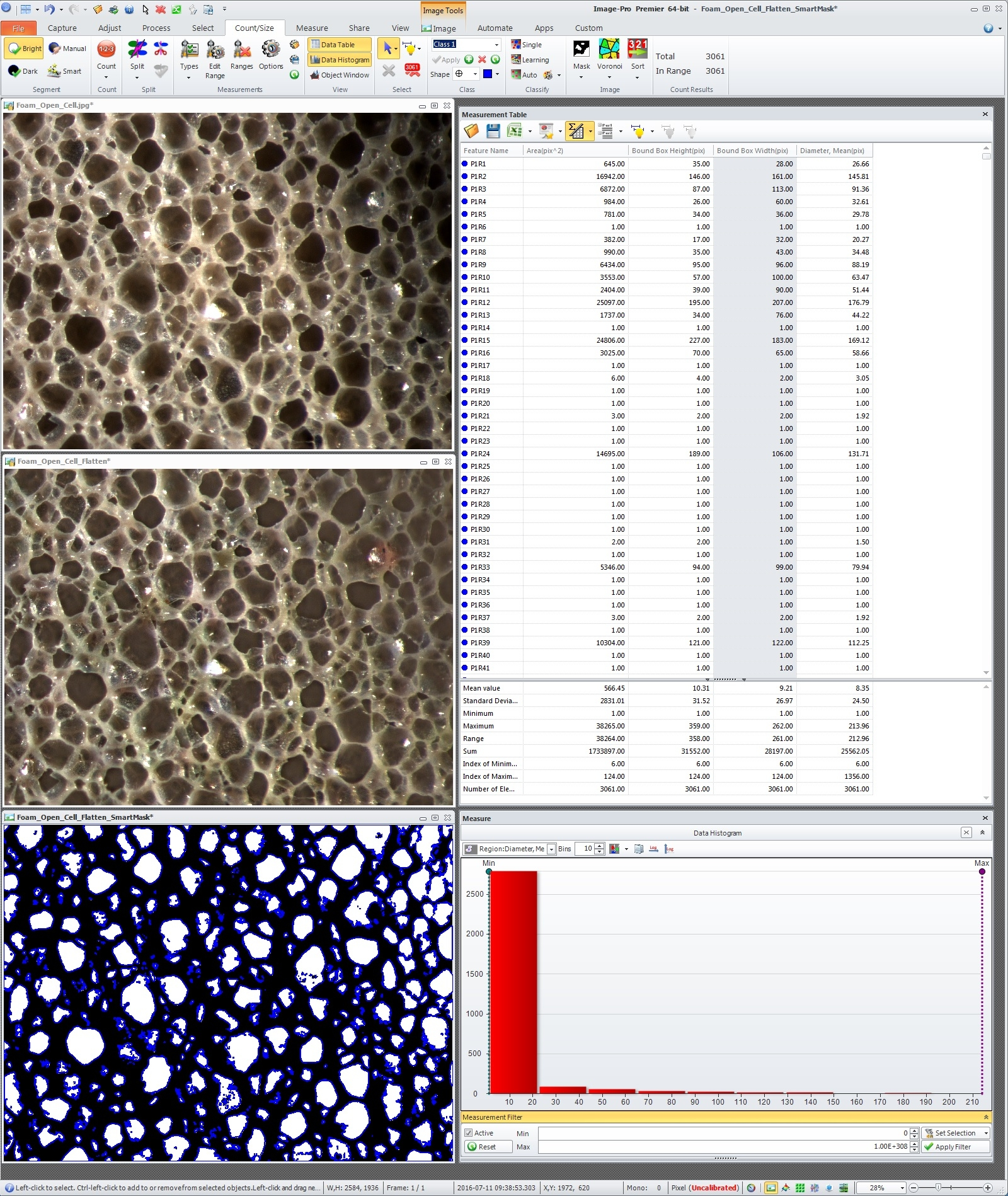# Particle size distribution in rubber modified system with TEM analysis

Hi

My field of applications is the study and the research of new multifasic polymeric systems rubber (EPR) embedded in a semicrystalline matrix. The quantitative morphological analysis was performed microtomed samples (in cryo modality under theTg conditions), obtaining the thin section useful for TEM analysis evaluation. Afterwards the image obtained was analysed in Image Analysis in order to obtain a quantitative particle-size distribution of the dispersed size (rubber). Assuming that the dispersed particles closely approach spherical shape, I d’ like to use a mathematical approach (or morphological Image Analysis macro) that permit me to convert the two-dimensional distribution of particle sections into the corresponding three-dimensional distribution of particle size. Moreover the approach should consider that the particles are intersected by a random plane can be measured by means TEM. The mathematical corrections, for converting the distribution of visible section diameters, should obtain a more realistic distribution.

Many thanks in advantage for all info that you give me regarding that topic.

Regards

M

• Hi Marco,

Image-Pro Premier can automatically measure multiple object parameters including area and diameters and also statistics (Max, Mean, StdDev,...), you can use these parameters to create derived measurements using custom formulas in Data Collector.

Regards,

Yuri
• Marco --

I located a FOAM EXAMPLE IMAGE on the INTERNET and did the following:

** FLATTEN
** SMART THRESHOLD
** MEASURE

The MEASUREMENTS that were made were:

** AREA
** BOUNDING BOX HEIGHT (MAX Y DIMENSION)
** BOUNDING BOX WIDTH (MAX X DIMENSION)
** MEAN DIAMETER

These MEASUREMENTS were made with NO CALIBRATION so everything is based solely on PIXELS (rather than MILLIMETERS or INCHES).  If the SAMPLE IMAGE is CALIBRATED, the MEASUREMENTS will be in the appropriate units like MILLIMETERS or INCHES.

The MEASUREMENTS can be seen INDIVIDUALLY and / or STATISTICALLY in the DATA TABLE / MEASUREMENT TABLE.

A GRAPHICAL PRESENTATION of the MEASUREMENTS can be seen in the DATA HISTOGRAM.

Please see the SCREEN CAPTURE in the JPG IMAGE FILE below.

The conclusions that can be drawn from these measurements are based on the SAMPLE and the SAMPLE COLLECTION.

Should a PROCEDURE like this provide the MEASUREMENTS that you need, the PROCEDURE can be automated using the PREMIER PROJECT WORKBENCH.

I hope this information is helpful.

-- Matt

*-*-*-*-*-*-*-*-*-*-*-*-*-*-*-*-*-*-*-*-*-*-*-*-*-*-*-*-*-*-*-*-*-*-*-*-*-*-*-*-*-• Hi,

many thx for your feedback, but I already know the possibility to obtain the measure of multiple object and I  did it. My question regarding the possibility to obtain a method  for converting the distribution of visible section diameters 2D in the corresponding 3D distribution of particle size (considering that the particles are intersected by a random plane). I'd like to receive some pratical examples or some ref of literature that explain how it do.

Regards

Marco

• Hi Marco,

You can simply google "stereology, spherical particle size" or "stereology saltykov method" to find documents describing 3D size distribution based on 2D section measurements.
Here is a couple of links:
https://www.ariel.ac.il/sites/conf/mmt/mmt-2006/Service_files/papers/Session_3/3-138_2d.pdf
http://www.ias-iss.org/ojs/IAS/article/viewFile/841/744

Regards,

Yuri
• Hi Yuri,

many thx for your advices, I have just read the first one of two and very interesting approach, I'm not a mathematical so if you have some practice example for me could be useful to understand how to apply that theory. Many thx in advantage.

Regards

Marco

PS

an example of 2D value found is ECD(mean)=200um

• Hi Marco,

If that is your field of applications, then you have to study what stereology is and basics of statistics. Stereology gives you tools to calculate parameters of spherical particles based on diameter distribution on 2D sections. Premier can provide you with the distribution of object diameters.

Let me know if you have specific questions about measurements.

Regards,

Yuri
• Hi Yuri,
many thx for your help and I would to looking for some examples that permit me to apply the theory/model on my applications or similar. Regarding the object distribution It's not a problem.
Regards

M